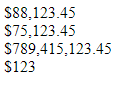Related Articles

# How to format a number as currency using CSS ?

• Last Updated : 03 Jun, 2020

Given a number and the task is to format the number of an HTML element as currency using CSS and a little bit of JavaScript. It is not possible to complete the task with pure CSS. A bit of JavaScript is needed for the parsing of the number to add the commas.

Approach: In the code, the CSS class `currSign` adds the currency sign (like ‘\$’) before the number. The JavaScript function `toLocaleString()` returns a string with a language-sensitive representation of the number.

Example:

 ```<``html``>`` ` `<``head``>``    ``<``meta` `name``=``"viewport"` `content``=``        ``"width=device-width, initial-scale=1"``>`` ` `    ````    ``<``style``>``        ``.currSign:before {``            ``content: '\$';``        ``}``    `````` ` `<``body``>``    ````    ``<``div` `class``=``"myDIV"``>88123.45``    ``<``div` `class``=``"myDIV"``>75123.45``    ``<``div` `class``=``"myDIV"``>789415123.45``    ``<``div` `class``=``"myDIV"``>123`` ` `    ````    ``<``script``>``        ``let x = document.querySelectorAll(".myDIV");``        ``for (let i = 0, len = x.length; i < ``len``; i++) {``            ``let ``num` `= ``Number``(x[i].innerHTML)``                      ``.toLocaleString('en');``            ``x[i]``.innerHTML` `= ``num``;``            ``x[i].classList.add("currSign");``        ``}``    `````` ` ``

Output:Attention reader! Don’t stop learning now. Get hold of all the important HTML concepts with the Web Design for Beginners | HTML  course.

My Personal Notes arrow_drop_up